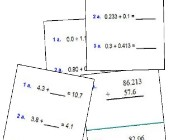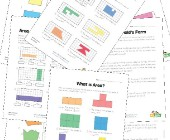Search

About 15 Search Results Matching Types of Worksheet, Worksheet Section, Generator, Generator Section, Type matching Worksheet Section, Grades matching 4th Grade, Similar to Spring Word Scramble Worksheet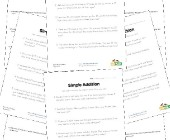Word Problem Worksheets

Help children develop their problem solving skills...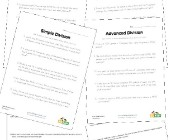Division Word Problems

Practice division and problem solving with these d...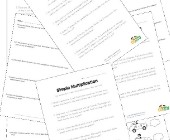Multiplication Word Problems

Practice multiplication and problem solving with t...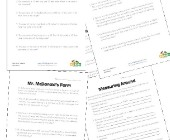Area and Perimeter Word Problems

This collection of area and perimeter worksheets c...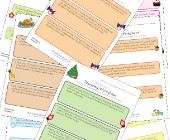Rounding Worksheets

Check out our collection of rounding worksheets in...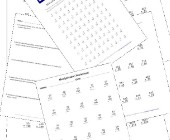Multiplication Worksheets for Kids

This set of math worksheets is geared towards help...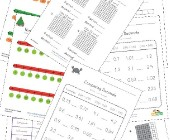Decimal Worksheets

This collection of free decimal worksheets will he...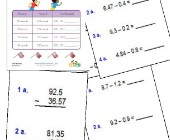Subtracting Decimals Worksheets

These worksheets will help children practice decim...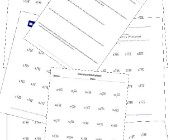Division Worksheets

Help kids learn division with this collection of m...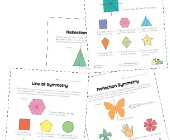Symmetry Worksheets

Help kids learn about the concept of symmetry with...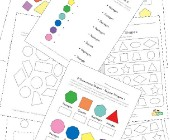Geometry Worksheets

Help kids learn the early basics of geometry inclu...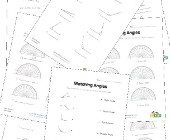Angles Worksheets

Help kids learn about angles with this collection ...